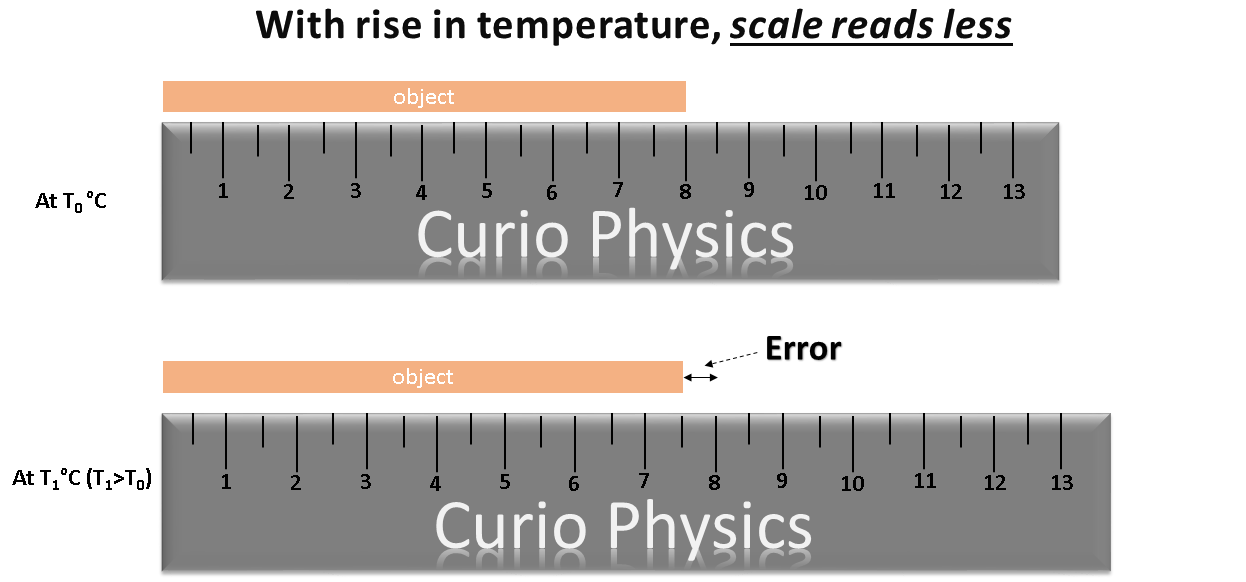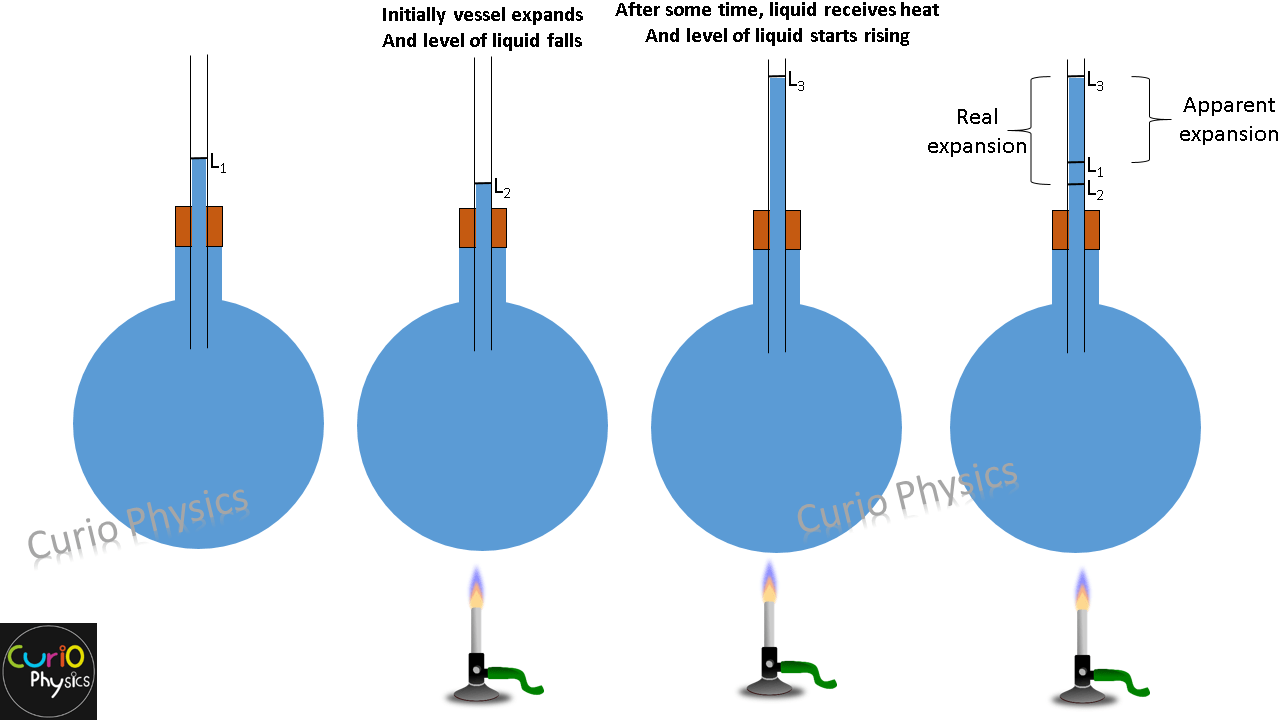# Error in Metal Scale Reading Due To Expansion and Contraction

### Error in Metal Scale Reading Due To Expansion and Contraction

Suppose we have a metal scale. As we know, with rise and fall in temperature, the length of metal scale changes and hence the distance between the graduations on the scale also changes. So with the change in temperature there arises error in the reading of a metal scale.

Error in Metal Scale Reading due to Rise in temperature :-

Let a metal scale gives correct reading at a temperature T0 ºC and the temperature at which we are measuring the length of an object is T1ºC(T1>T0). With the rise in temperature the distance between any two scale divisions increases in the ratio 1: (1 + αΔT).

Here ΔT = T1 – T0    and α = coefficient of linear expansion of the material of the scale.

If the scale is measuring the length of an object as L cm, then its actual reading must be L(1 + αΔT)cm and hence a correction of  +LαΔT should be applied.In above figure the actual length of the object is 8 cm, but as the temperature rises to T1ºC , the length of the scale increases and the scale reads less i.e., 7.5 cm.

Here

Error = M.V. (measured value)  –  T.V. (True value) =  7.5cm – 8cm = -0.5 cm

Correction = +0.5 cm

Error in Metal Scale Reading due to Fall in temperature :-

As the temperature falls, the length of the metal scale reduces and the scale reads more.

Let the temperature be T2ºC(T2<T0).

With the fall in temperature the distance between any two scale divisions decreases in the ratio 1: (1 – αΔT).

Here ΔT = T0 – T2    and α = coefficient of linear expansion of the material of the scale.

If the scale is measuring the length of an object as L cm, then its actual reading must be L(1 – αΔT)cm and hence a correction of  -LαΔT should be applied.In above figure the actual length of the object is 8 cm, but as the temperature falls to T2ºC , the length of the scale decreases and the scale reads more i.e., 8.5 cm.

Here

Error = M.V. (measured value)  –  T.V. (True value) =  8.5cm – 8cm = +0.5 cm

Correction = -0.5 cm

Example

A meter scale is made of steel and measures correct length at 16ºC. what will be the percentage error if the scale is used

• on a summer day when temperature is 46ºC  and
• on a winter day when the temperature is 6ºC ?

Coefficient of linear expansion of steel is 11×10-6 ºC-1.

Solution.

On a summer day, the scale will read less than the actual length. Hence, the percentage error will be :-

percentage error = –(Δl/l) ×100

⇒    percentage error = -(αΔT) ×100

⇒    percentage error = -( 11×10-6 ) ×(46-16)×100

⇒    percentage error = -0.033%

On a winter day, the scale will read more than the actual length. Hence, the percentage error will be :-

percentage error = +(Δl/l) ×100

⇒    percentage error = +(αΔT) ×100

⇒    percentage error = +( 11×10-6 ) ×(16-6)×100

⇒    percentage error = +0.011%